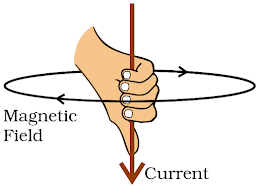# MAGNETIC EFFECTS OF ELECTRIC CURRENT

MAGNETIC FIELD DUE TO A CURRENT-CARRYING CONDUCTOR

# Experiment to find the direction of the field:

• Take a long straight copper wire, 2 or 3 cells of 1.5 V each, and a plug key. Connect them in series.
• Place copper wire parallel to and over a compass needle.
• If the current flows from north to south, the north pole of the compass needle moves towards the east.
• If the current flows from south to north, the needle moves in opposite direction (towards west).
• It means that the direction of magnetic field produced by the electric current is also reversed.

Magnetic Field due to a Current through a Straight Conductor

Insert a straight thick copper wire through the centre, normal to the plane of a rectangular cardboard.

Connect the copper wire vertically between the points X & Y, in series with a battery (12 V), a variable resistance (or rheostat), an ammeter (0–5 A) and a plug key.

Sprinkle some iron filings uniformly on the cardboard.

Close the key. Gently tap the cardboard a few times. The iron filings align as a pattern of concentric circles. They represent magnetic field lines around the copper wire.

(a) A pattern of concentric circles. The arrows show the direction of the field lines. (b) A close up of the pattern.

Place a compass at a point (say P) over a circle. The direction of the north pole of the compass needle gives the direction of the field lines at P.

The direction of magnetic field lines is reversed if the direction of current through the copper wire is reversed.

If we vary the current in the copper wire, the deflection in the needle also changes. If the current is increased, the deflection also increases. Thus, the magnitude of magnetic field produced at a given point increases as the current through the wire increases.

If the compass is placed at a farther point (say Q) from the conducting wire, deflection in the needle decreases. Thus, magnetic field produced by current in the conductor decreases as the distance increases (inversely proportional). The concentric circles representing magnetic field around a current-carrying straight wire become larger as we move away from it.

Right-Hand Thumb Rule

It is an easy way to find the direction of magnetic field associated with a current-carrying conductor.Right-hand thumb Rule

Imagine a current-carrying straight conductor is held in right hand such that the thumb points towards the direction of current. Then the fingers will wrap around the conductor in the direction of the magnetic field lines. This is known as the right-hand thumb rule (Maxwell’s corkscrew rule).

Problem: A current through a horizontal power line flows in east to west direction. What is the direction of magnetic field at a point directly below it and at a point directly above it?

Solution

Applying the right-hand thumb rule, magnetic field (at any point below or above the wire) turns clockwise in a plane perpendicular to the wire, when viewed from east end, and anti-clockwise, when viewed from the west end.

Magnetic Field due to a Current through a Circular Loop

Suppose a straight wire is bent to form a circular loop and a current is passed through it. Here, at every point of circular loop, the concentric circles around it become larger as the distance from the wire increases.

At the centre of the circular loop, the arcs of these big circles appear as straight lines. Every point on the wire give rise to the magnetic field appearing as straight lines at the centre of loop. By applying the right-hand rule, every section of the wire contributes to the magnetic field lines in same direction within the loop.

The magnetic field produced by a current-carrying wire at a given point depends directly on the current passing through it. So, for a circular coil with n turns, the field produced is n times as large as that produced by a single turn. This is because the current in each circular turn has the same direction, and the field due to each turn then just adds up.

Experiment:

•  Take a rectangular cardboard having two holes.
•   Insert a circular coil having large number of turns through them, normal to the plane of the cardboard.
•   Connect the ends of the coil in series with a battery, a key and a rheostat.
•   Sprinkle iron filings uniformly on the cardboard.
•   Plug the key and tap the cardboard gently a few times.
•   Concentric circle patterns of the iron filings emerge on the cardboard. At the centre, it appears as straight line.

Magnetic Field due to a Current in a Solenoid

A solenoid is a coil of many circular turns of insulated copper wire wrapped closely in the shape of a cylinder.

The pattern of the magnetic field lines around a current-carrying solenoid looks similar to the pattern of the field around a bar magnet.

One end of the solenoid behaves as a magnetic north pole and the other as the south pole. The field lines inside the solenoid are in the form of parallel straight lines. This indicates that the magnetic field is same (uniform) at all points inside the solenoid.

If a magnetic material (e.g. soft iron) is placed inside a current-carrying solenoid, it becomes magnetised. The magnet so formed is called an electromagnet.